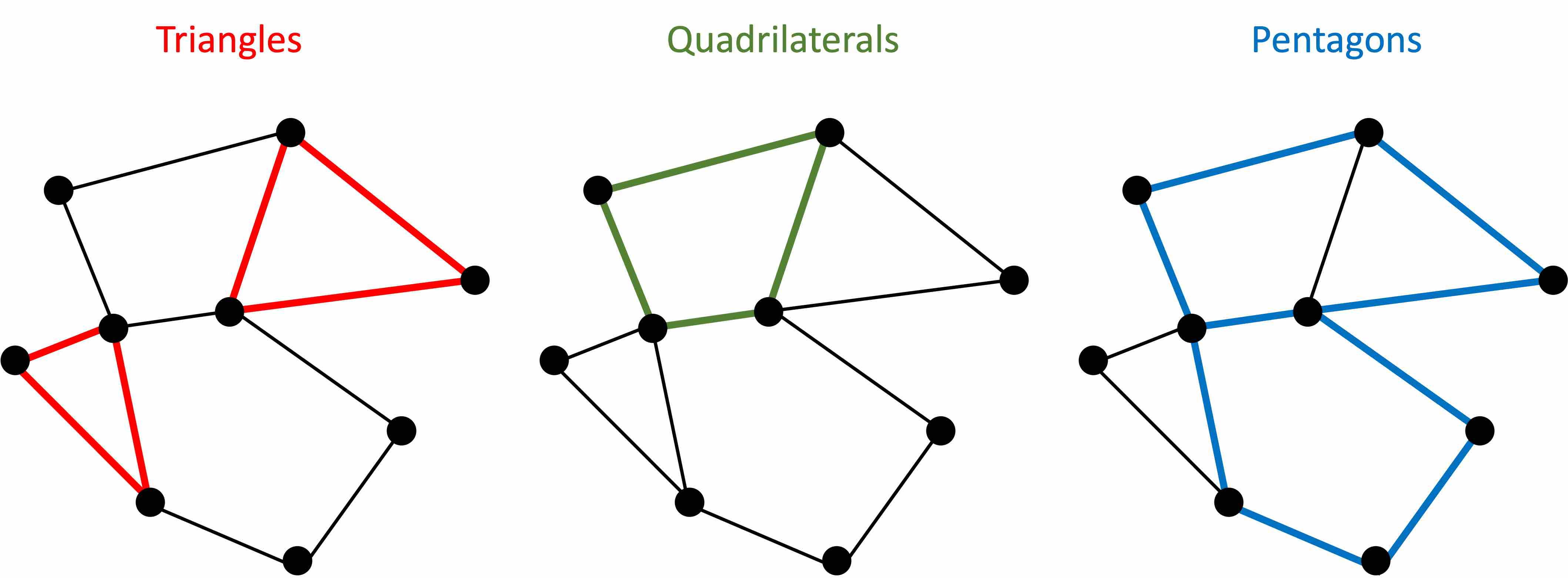## Motivation

Last week I got curious about counting triangles in node graphs after stumbling upon an elegant method. See my post Counting Triangles in Node Graphs for more details.

But it got me thinking: what about counting rectangles? Technically, I should say “quadrilaterals” since what I really mean is any 4-sided shape. It turned out not to be not quite so simple as counting triangles, but I found a way!

But then it got me thinking: what about counting pentagons? It turned out to be even harder than counting quadrilaterals, but I found a way!

But then it got me thinking: what about counting hexagons? It turned out to be even harder than counting pentagons, so I thought, “Eh, I’ll think about it later.”

Be that as it may, here I’ll describe my generalized solution for triangles, quadrilaterals, and pentagons below, which includes some nifty plotting work to help “prove” the accuracy of the solution.

## Review of Counting Triangles

When counting triangles, here is the elegant python solution that first got me excited

Suppose `S` is a numpy array of the square matrix representation of an undirected node graph (with no self edges), then

``````triangles_count = S.dot(S).dot(S).trace() / 6
``````

Crucial to this logic is S • S • S or S3, which has the following characteristics: each `(i, j)` represents the number of ways you can cross exactly 3 edges when you start at `i` and end at `j`.

So when it comes to triangles, you can just add up all the diagonal of S3 and divide by 6 to get the count of triangles. Once again, check out my post Counting Triangles in Node Graphs for a deeper explanation.

## Why It’s Harder to Count Quadrilaterals and Pentagons Than Triangles

My initial hope that was that there would be a simple way to extend the solution to the triangles problem to 4-sided shapes involving the trace of S4. To write it in python, my hope that there was some `k` for which `quads_count = S.dot(S).dot(S).dot(S).trace() / k`. Unfortunately, it turned out not to be the case.

First consider the count of paths on diagonal of S3: each path is guaranteed to be a triangle. You simply can’t pass through three edges AND end up at the same place that you started from UNLESS you travel in a triangle.

With S4, unfortunately, you are not guaranteed that the paths on the diagonal will represent quadrilaterals. As the simplest example, suppose there is an edge connecting `A` -> `B`. Well, wouldn’t you know, if you go from `A` to `B` and back to `A` and back to `B` and finally back to `A`, you will have successfully traversed 4 edges and ended up where you started. As this counterexample shows, only looking at the trace of S4 isn’t enough to accurately identifying quadrilaterals, seeing as the count of 4-paths on the diagonal will include many paths that aren’t quadrilaterals. The same is also true when trying to count pentagons from the diagonal of S5.

I won’t go into all the details here, so take a good look at the source code in the Jupyter notebook below for my solution to those two cases.

## Code Examples

Be sure to check out the plotting that visually identifies the triangles, quadrilaterals, and pentagons in a node graph.

Here is a link to a full-page view of the notebook. Or you can download a copy of the .ipynb file here.